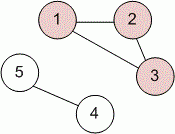favorite We need a little bit of your help to keep things running, click on this banner to learn more
Problems

# Depth Search

The undirected unweighted graph with one selected vertex is given. Find the number of vertices in the connected component where the selected vertex (including the selected vertex).

#### Input

The first line contains the number of vertices n and the selected vertex s (1sn100). In the next n lines given n integers - the adjacency matrix of a graph, where "0" means the absence of an edge, and "1" means the presence of an edge. It is guaranteed that the main diagonal of the matrix contains zeros.

#### Output

Print the desired number of vertices.Time limit 1 second
Memory limit 128 MiB
Input example #1
```5 1
0 1 1 0 0
1 0 1 0 0
1 1 0 0 0
0 0 0 0 1
0 0 0 1 0
```
Output example #1
```3
```